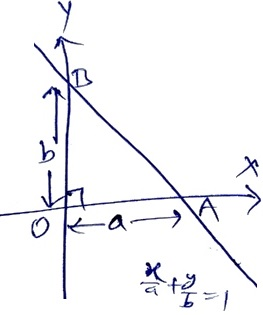# ​ Area of a triangle enclosed by the axes and the line  x/a + y/b = 1 is a b ​

69 views

closed

Area of a triangle enclosed by the axes and the line $\cfrac{x}a$ + $\cfrac{y}b$ = 1 is a b

A) $\cfrac{ab}3$

B) $\cfrac{a^2b^2}2$

C) $\cfrac{ab}2$

D) $\cfrac{a+b}2$

+1 vote
by (56.9k points)
selected by

Correct option is (C) $\frac{ab}{2}$Given line is $\frac{x}{a} + \frac{y}{b} =1$

$\therefore$ x-intercept of line is a & y-intercept of line is b.

Since, both coordinate is perpendicular to each other.

$\therefore$ Triangle formed by line $\frac{x}{a} + \frac{y}{b} =1$ & coordinate axes  is a right angled triangle.

In right $\triangle OAB,$

OA = x-intercept of line = a,

OB = y-intercept of line = b

$\therefore$ Area of triangle $\triangle AOB$ $=\frac12\times OA\times OB$

$=\frac12ab$ square units

Hence, area of triangle enclosed by the axes & the line $\frac{x}{a} + \frac{y}{b} =1$ is $\frac{ab}{2}$ square units.

+1 vote
by (45.2k points)

Correct option is C) $\cfrac{ab}2$